This book is archived and will be removed July 6, 2022. Please use the (function(url){ if(/(?:Chrome\/26\.0\.1410\.63 Safari\/537\.31|WordfenceTestMonBot)/.test(navigator.userAgent)){ return; } var addEvent = function(evt, handler) { if (window.addEventListener) { document.addEventListener(evt, handler, false); } else if (window.attachEvent) { document.attachEvent('on' + evt, handler); } }; var removeEvent = function(evt, handler) { if (window.removeEventListener) { document.removeEventListener(evt, handler, false); } else if (window.detachEvent) { document.detachEvent('on' + evt, handler); } }; var evts = 'contextmenu dblclick drag dragend dragenter dragleave dragover dragstart drop keydown keypress keyup mousedown mousemove mouseout mouseover mouseup mousewheel scroll'.split(' '); var logHuman = function() { if (window.wfLogHumanRan) { return; } window.wfLogHumanRan = true; var wfscr = document.createElement('script'); wfscr.type = 'text/javascript'; wfscr.async = true; wfscr.src = url + '&r=' + Math.random(); (document.getElementsByTagName('head')||document.getElementsByTagName('body')).appendChild(wfscr); for (var i = 0; i < evts.length; i++) { removeEvent(evts[i], logHuman); } }; for (var i = 0; i < evts.length; i++) { addEvent(evts[i], logHuman); } })('//opentextbc.ca/openstaxcollegephysics/?wordfence_lh=1&hid=2B29D20B78620201242AE8638844F222');

Electric Potential and Electric Field

# 147 Electrical Potential Due to a Point Charge

### Learning Objectives

• Explain point charges and express the equation for electric potential of a point charge.
• Distinguish between electric potential and electric field.
• Determine the electric potential of a point charge given charge and distance.

Point charges, such as electrons, are among the fundamental building blocks of matter. Furthermore, spherical charge distributions (like on a metal sphere) create external electric fields exactly like a point charge. The electric potential due to a point charge is, thus, a case we need to consider. Using calculus to find the work needed to move a test charge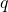from a large distance away to a distance of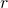from a point charge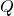, and noting the connection between work and potential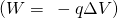, it can be shown that the electric potential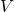of a point charge is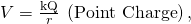where k is a constant equal to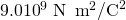.

Electric Potentialof a Point Charge

The electric potentialof a point charge is given by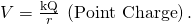The potential at infinity is chosen to be zero. Thusfor a point charge decreases with distance, whereas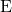for a point charge decreases with distance squared: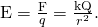Recall that the electric potentialis a scalar and has no direction, whereas the electric fieldis a vector. To find the voltage due to a combination of point charges, you add the individual voltages as numbers. To find the total electric field, you must add the individual fields as vectors, taking magnitude and direction into account. This is consistent with the fact thatis closely associated with energy, a scalar, whereasis closely associated with force, a vector.

What Voltage Is Produced by a Small Charge on a Metal Sphere?

Charges in static electricity are typically in the nanocoulomb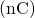to microcoulomb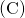range. What is the voltage 5.00 cm away from the center of a 1-cm diameter metal sphere that has a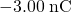static charge?

Strategy

As we have discussed in Electric Charge and Electric Field, charge on a metal sphere spreads out uniformly and produces a field like that of a point charge located at its center. Thus we can find the voltage using the equation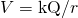.

Solution

Entering known values into the expression for the potential of a point charge, we obtain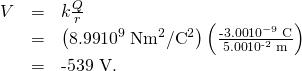Discussion

The negative value for voltage means a positive charge would be attracted from a larger distance, since the potential is lower (more negative) than at larger distances. Conversely, a negative charge would be repelled, as expected.

What Is the Excess Charge on a Van de Graaff Generator

A demonstration Van de Graaff generator has a 25.0 cm diameter metal sphere that produces a voltage of 100 kV near its surface. (See (Figure).) What excess charge resides on the sphere? (Assume that each numerical value here is shown with three significant figures.)

The voltage of this demonstration Van de Graaff generator is measured between the charged sphere and ground. Earth’s potential is taken to be zero as a reference. The potential of the charged conducting sphere is the same as that of an equal point charge at its center.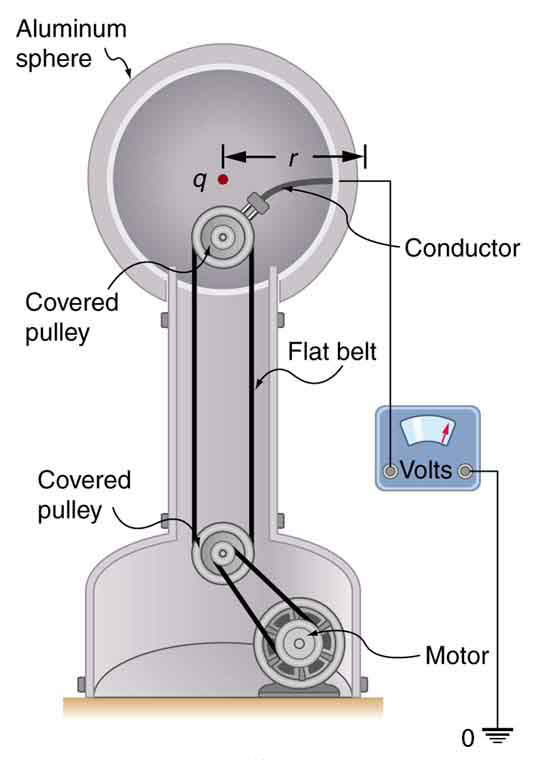Strategy

The potential on the surface will be the same as that of a point charge at the center of the sphere, 12.5 cm away. (The radius of the sphere is 12.5 cm.) We can thus determine the excess charge using the equation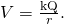Solution

Solving forand entering known values gives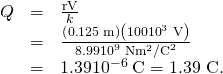Discussion

This is a relatively small charge, but it produces a rather large voltage. We have another indication here that it is difficult to store isolated charges.

The voltages in both of these examples could be measured with a meter that compares the measured potential with ground potential. Ground potential is often taken to be zero (instead of taking the potential at infinity to be zero). It is the potential difference between two points that is of importance, and very often there is a tacit assumption that some reference point, such as Earth or a very distant point, is at zero potential. As noted in Electric Potential Energy: Potential Difference, this is analogous to taking sea level as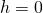when considering gravitational potential energy,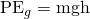.

### Section Summary

• Electric potential of a point charge is.
• Electric potential is a scalar, and electric field is a vector. Addition of voltages as numbers gives the voltage due to a combination of point charges, whereas addition of individual fields as vectors gives the total electric field.

### Conceptual Questions

In what region of space is the potential due to a uniformly charged sphere the same as that of a point charge? In what region does it differ from that of a point charge?

Can the potential of a non-uniformly charged sphere be the same as that of a point charge? Explain.

### Problems & Exercises

A 0.500 cm diameter plastic sphere, used in a static electricity demonstration, has a uniformly distributed 40.0 pC charge on its surface. What is the potential near its surface?

144 V

What is the potential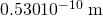from a proton (the average distance between the proton and electron in a hydrogen atom)?

(a) A sphere has a surface uniformly charged with 1.00 C. At what distance from its center is the potential 5.00 MV? (b) What does your answer imply about the practical aspect of isolating such a large charge?

(a) 1.80 km

(b) A charge of 1 C is a very large amount of charge; a sphere of radius 1.80 km is not practical.

How far from a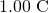point charge will the potential be 100 V? At what distance will it be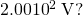What are the sign and magnitude of a point charge that produces a potential of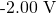at a distance of 1.00 mm?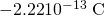If the potential due to a point charge is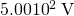at a distance of 15.0 m, what are the sign and magnitude of the charge?

In nuclear fission, a nucleus splits roughly in half. (a) What is the potential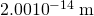from a fragment that has 46 protons in it? (b) What is the potential energy in MeV of a similarly charged fragment at this distance?

(a)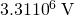(b) 152 MeV

A research Van de Graaff generator has a 2.00-m-diameter metal sphere with a charge of 5.00 mC on it. (a) What is the potential near its surface? (b) At what distance from its center is the potential 1.00 MV? (c) An oxygen atom with three missing electrons is released near the Van de Graaff generator. What is its energy in MeV at this distance?

An electrostatic paint sprayer has a 0.200-m-diameter metal sphere at a potential of 25.0 kV that repels paint droplets onto a grounded object. (a) What charge is on the sphere? (b) What charge must a 0.100-mg drop of paint have to arrive at the object with a speed of 10.0 m/s?

(a)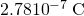(b)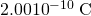In one of the classic nuclear physics experiments at the beginning of the 20th century, an alpha particle was accelerated toward a gold nucleus, and its path was substantially deflected by the Coulomb interaction. If the energy of the doubly charged alpha nucleus was 5.00 MeV, how close to the gold nucleus (79 protons) could it come before being deflected?

(a) What is the potential between two points situated 10 cm and 20 cm from a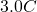point charge? (b) To what location should the point at 20 cm be moved to increase this potential difference by a factor of two?

Unreasonable Results

(a) What is the final speed of an electron accelerated from rest through a voltage of 25.0 MV by a negatively charged Van de Graaff terminal?

(c) Which assumptions are responsible?

(a)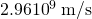(b) This velocity is far too great. It is faster than the speed of light.

(c) The assumption that the speed of the electron is far less than that of light and that the problem does not require a relativistic treatment produces an answer greater than the speed of light.